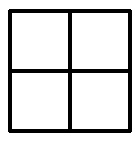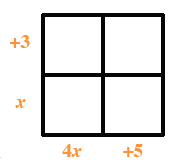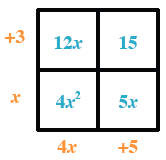### Home > CC4 > Chapter 3 > Lesson 3.3.1 > Problem3-117

3-117.

Multiply each of the following expressions. Show all of your work. Homework Help ✎

1. $(x+3)(4x+5)$

Use a generic rectangle to multiply.

Put the products on the outside of the rectangle.

Use length times width to find the areas of the small rectangles.

Write the total area as a sum.4x2 + 12x + 5x + 15

4x2 + 17x + 15

2. $(−2x^2−4x)(3x+4)$

Follow the steps in part (a).

−6x3 − 20x2 − 16x

3. $(3y−8)(−x+y)$

Follow the steps in part (a).

4. $(y−4)(3x+5y−2)$

Follow the steps in part (a).

3xy + 5y2 − 22y − 12x + 8